# RS Aggarwal Class 7 Solutions Chapter 10 Percentage Ex 10A

## RS Aggarwal Class 7 Solutions Chapter 10 Percentage Ex 10A

These Solutions are part of RS Aggarwal Solutions Class 7. Here we have given RS Aggarwal Solutions Class 7 Chapter 10 Percentage Ex 10A.

Other Exercises

Question 1.
Solution: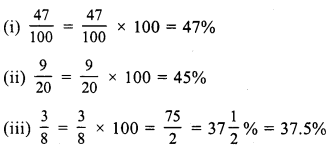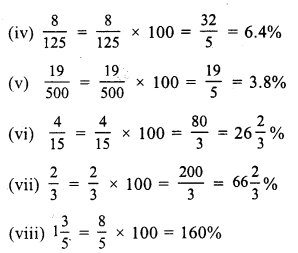Question 2.
Solution: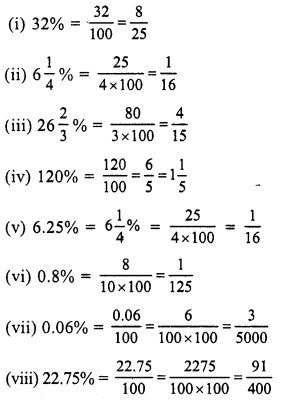Question 3.
Solution: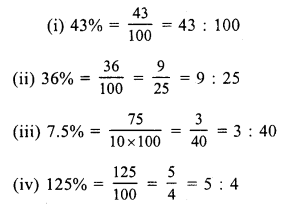Question 4.
Solution: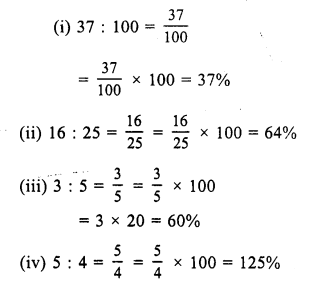Question 5.
Solution: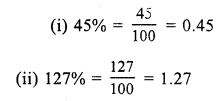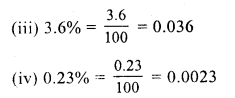Question 6.
Solution: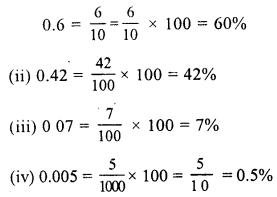Question 7.
Solution: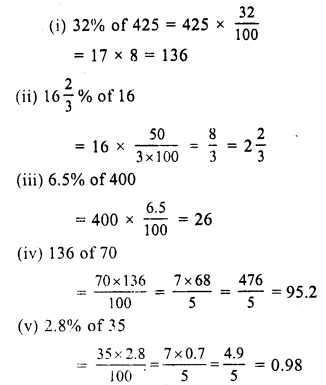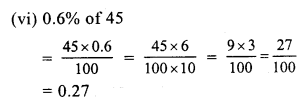Question 8.
Solution: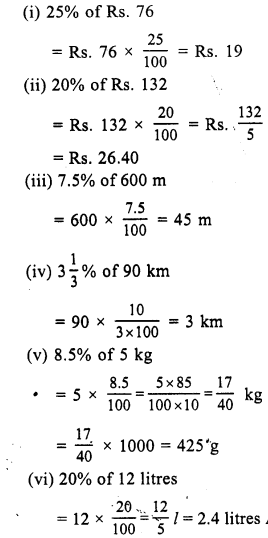Question 9.
Solution:
Let x is the required number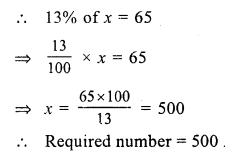Question 10.
Solution:
Let x be the required number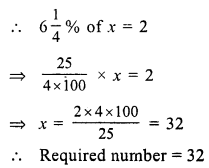Question 11.
Solution:
10 % of Rs. 90 = 90 x $$\frac { 10 }{ 100 }$$ = Rs. 9
Required amount = Rs. 90 + Rs. 9 = Rs. 99

Question 12.
Solution:
20 % of Rs. 60 = $$\frac { 60 x 20 }{ 100 }$$ = 12
Required amount = Rs. 60 – 12 = Rs. 48

Question 13.
Solution:
3 % of x = 9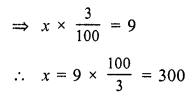Question 14.
Solution:
12.5 % of x = 6
⇒ x x $$\frac { 12.5 }{ 100 }$$ = 6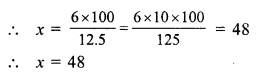Question 15.
Solution:
Let x % of 84 = 14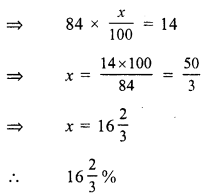Question 16.
Solution: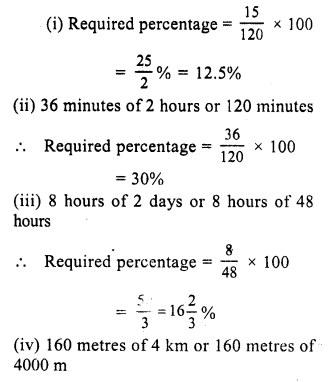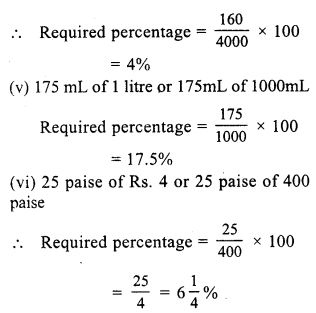Hope given RS Aggarwal Solutions Class 7 Chapter 10 Percentage Ex 10A are helpful to complete your math homework.

If you have any doubts, please comment below. Learn Insta try to provide online math tutoring for you.